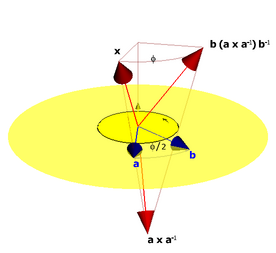# Quaternions and Geometric Algebra

Amazon.com automatically deduced from its sales and search figures that many who are interested in quaternions are also exploring geometric algebra. We created this page to explain the relationship between the two, and to indicate how our book can help you understand and visualize quaternions, and extend their applicability considerably.

Traditionally, quaternions are thought of as an extension of complex numbers to 3-D, with three instead of one imaginary parts i, j, k, each of which squares to -1.Figure 10-5: Interpolating between orientations.
In computer graphics, unit quaternions are used to represent orientations. To transform a vector x from a standard orientation to the orientation represented by the quaternion q, one multiplies on the left and divides on the right by the quaternion: qx/q. By interpolating between two unit quaternions and then applying the interpolated quaternion to a vector, the orientation of the vector moves smoothly from one orientation to another orientation. To transform an object, one transforms all the elements of a coordinate frame, and performs a change of basis at each step to transform the object.

However, there are a variety of issues with quaternions. Aside from their mysterious, non-geometric manifestation, using quaternions to interpolate between two orientations often requires transforming to and from the quaternion representation; quaternions can represent only rotations around a line through the origin; and quaternions rotate only vectors and not other objects such as lines or planes.Figure 7-2: Rotation of the vector x through the rotor ba. The bivector part of the rotor (strong yellow) represents the plane of rotation, and the angle between the two vectors is half the rotation angle.
In geometric algebra, rotors in 3-D Euclidean space are closely related to quaternions. The correspondence between the two is discussed in Chapter 7 of our book.

Any rotation is a double reflection in a pair of vectors, as illustrated in Figure 7-2 on the right. In this figure, the red vector x is reflected first in the blue vector a, and then in the blue vector b. The result is that x is rotated over an angle φ, which is twice the angle between a and b.

In geometric algebra, you can use a unit vector a as a reflection operator by employing it in a sandwiching product: a x/a reflects the vector x in the line of a.

It then follows from the figure that the geometric product of two unit vectors R = b a fully encodes the rotation as a double reflection. R is called a rotor, and it is a regular element of the real algebra. The rotation of any object O by the rotor R is performed the same way as quaternions: RO/R. Quaternions are just one special case of rotors, i.e., quaternions are rotors for a 3-D Euclidean space. The i, j, k coordinates of a quaternion are just the coordinates of the bivector part of the rotor, which encodes the rotation plane (drawn as the yellow disc in the figure on the right). In geometric algebra, the square of a real unit plane like i happens to be -1, and that is why rotors and quaternions remind us of complex numbers. Emphasizing this relationship to complex numbers (as quaternion treatments typically do) makes them needlessly hard to understand and visualize.

Interpolation between two rotors is explored in Chapter 10 of our book, and is as simple as you would multiplicatively interpolate between two real numbers x and y by x(x-1y)α = x exp(α log(x-1y)), with α varying from 0 to 1:

``````
// partially interpolate between 'src' and 'dst', as determined by 'alpha'
rotor interpolateRotor(const rotor &src, const rotor &dst, mv::Float alpha) {
// return src * exp(alpha * log(dst * inverse(src)));
return _rotor(src * exp(_bivector(alpha * log(_rotor(inverse(src) * dst)))));
}

``````
That is the nice thing about geometric algebra: you can compute with geometrical operators and objects almost as if they were numbers. This function was taken from programming example 10-1; the exponential and logarithm functions are present in the freely downloadable GA Sandbox library and described in detail in our book (Chapters 7 and 10).

#### Bottom Line

While they are in many ways similar, there are several advantages of using rotors in a geometric algebra setting over using quaternions:
• Rotors are natural and non-mysterious in geometric algebra and easily understood as the encoding of a double reflection.
• In geometric algebra, a rotor and the objects it acts on live in the same space. This eliminates the need to change representations and to encode new data structures and methods (which is required when augmenting linear algebra with quaternions).
• A rotor is universally applicable to any element of the algebra, not just vectors and other quaternions, but also lines, planes, circles, spheres, rays, and so on.
• In the conformal model of Euclidean geometry (Chapters 13 to 16), rotors allow us to encode rotation, translation and scaling in a single element of the algebra, universally acting on any element. In particular, this means that rotors can represent rotations around an arbitary axis, whereas quaternions are limited to an axis through the origin.
• We can interpolate between rotor-encoded transformations using the same code as shown above (although the logarithm for general rotors still needs to be developed, our book shows how to interpolate any scaled rigid body motion in 3-D in Chapter 16).
Chapter 16, Programming Example 4: Interpolation of the rotor for a scaled rigid body motion, applied to a green circle, and a small red sphere.